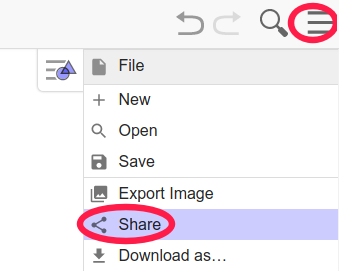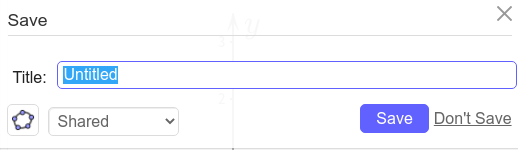# GeoGebra必学必用经验

GeoGebra(官网 https://geogebra.org/ , 音译: 几何不难)是一款开源跨平台的非商用免费软件, 一定要注意商用无价, 当心被坑. GeoGebra有三个版本, 分别是版本5, 版本6和网页版, 点我查看三者的区别.

• 下载地址 https://kz16.top/ggbppt.7z (体积不到10M, 免费开源无广告, 仅用于学习, 请勿商用, 跨平台, 解压可用, 推荐用 https://7-zip.org/ 解压), 外加的功能可以通过H键隐藏, 激光笔或上下方向键翻页

• 注: ggbppt是基于GeoGebra的离线网页版开发的多页实用模板, 底层依旧用得是GeoGebra官网提供的网页版, 只是额外增加了几个实用按钮, 并自己设置了一个实用模板. 下了离线包后, 可以无需安装GeoGebra桌面版.

# 1. 必用操作

## 1.1. 导入ggb文件

• 紧按鼠标左键, 直接把ggb文件拖入到画图的界面中

## 1.2. 保存ggb文件

• 界面右上角三字形状的图标--->Download as--->ggb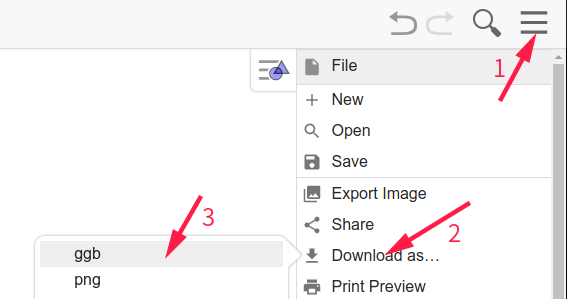• 其实最简单的办法是点击页面 https://kz16.top/geogebra/ggbppt.html 最上方的 导出在线 按钮即可, 保不保存ggb文件无所谓. 当然也可以导出离线 , 这需要下载离线包, 并且要把离线文件放到离线包中.

## 1.4. 导入图片

• 法1: 紧按鼠标左键, 直接把图片拖入到画图的界面中

• 法2: 点击最上边滑动条那个图标--->Image--->Choose File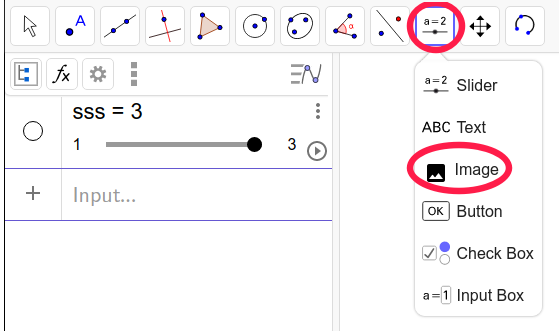## 1.5. 导出透明svg矢量图

• 在命令行中输入下列代码再回车即可
ExportImage("filename","image.svg","type","svg")
下面代码可以得到不透明svg矢量图
ExportImage("filename","image.svg")

• 如果想要得到固定区域的svg图有两种办法:

1. 右键框选所要导出的区域
2. 先输入代码
SetPerspective("(G/D)(A/P)"
拖动边框调整下界面大小, 再输入下面代码回车
ExportImage("filename","image.svg","type","svg")
• 推荐用inkscape把svg转化为pdf文件

## 1.6. 提取ggb中被插入的图片

• 只需要把ggb文件用解压软件解压即可, 推荐用免费无广告的 https://7-zip.org/ 解压

# 2. 必学技巧

## 2.1. 常用小技巧

• 绘图的过程中, 点了绘图小图标工具后一定要记得按Esc

• 鼠标右键可设置属性, 或者框选多个对象

• Shift或者Ctrl可以一次性选择多个对象

• 尽量用代码画图, 推荐代码用markdown编辑器保存起来, 参考 https://kz16.top/md/

• 对不熟悉的指令, 可以用必应或者谷歌搜索, 搜索的词条为
geogebra 指令名, 例如geogebra zip, 一般第一条就会得到指令解释

## 2.2. 循环与求和

### 2.2.1. 序列循环zip

• 推荐用zip, 完全没必要用sequence

例如得到列表{1,4,9,16,25,36}可以这样写

zip(k^2,k,1..6)

### 2.2.2. 迭代Iteration

• IterationList
iterationList(p*10+1, p, {1}, 2)
结果为 {1, 11, 111}

斐波那契数列
iterationList(b+a, a, b, {1, 1}, 5)
结果为 {1,1,2,3,5,8}

• Iteration
iterationList(p*10+1, p, {1}, 2)
结果为{111}

### 2.2.3. 序列求和Sum

• 求和要注意大写, 求列表
{1,4,9,16,25,36}的和
Sum(k^2,k,1,6)

zip括号中的1..6是用两点隔开的
Sum括号中的1,6是用逗号隔开的

## 2.3. 判断

f(x)=if(x<0,-1,x<1,x,1)

curve((2cos(t), sin(t)),t,0,pi/2)

## 2.4. 列表

• 1..4得到结果{1,2,3,4}

• 合并join, 展平flatten 可分别看例子
join({1},{2,{3}})得到{1,2,{3}}
flatten({{1},{2,{3}}})得到{1,2,3}

• a(1)element(a, 1)表示列表a的第一个元素, a(-1) 表示列表a的最后一个元素, element(a, -1)会报错

• take(a,2,4)表示取列表a的第2,3,4号索引, 例如
take(1..6,2,4)得到2,3,4

• Union(a,b)表示a,b的并, Intersection(a,b)表示a,b的交

• 属于符号∈完全和数学一致

• 对列表循环, 通常用zip函数, 例如得到列表a={1,3,1,4}的平方, 可以这样
zip(k^2,k,a) 也可以直接a^2 结果都是{1,9,1,6}

• 同时对多个列表循环, 例如计算2个列表的和

a={1,2,3}
b={4,5,6}
zip(ak+bk,ak,a,bk,b)


上面最后一步当然也可以直接写成a+b

• {1,2}*3结果为{3,6}

• {1,2}+{3,4}结果为{4,6}

• ln({1,e,e^2})结果为{0,1,2}

• ... 其余的可自己去尝试

## 2.5. 向量与点

• A=(1,2), 则x(A)=1, y(A)=2

• A=(1,2), B=(3,0), 则它们的中点为(A+B)/2

• 用分号隔开则表示极坐标, 例如
B=(1;pi/3)

## 2.6. 复数

Z=1+3i

## 2.7. 插入特殊的标记对象

• 制作透明花括号的svg矢量图片
• t=text("\overbrace{\qquad\qquad}",(0,0),true,true)
• 右键框选花括号图片, 并输入下面代码, 回车
ExportImage("filename","image.svg","type","svg")
• 导入上一步的花括号图片image.svg, 默认导入的图片为辅助对象, 第一个图名字为pic1
• 接下来就是控制图片的大小, 任意绘制2个点A,B, 并且
C=A+i (B-A)/abs(A-B)*aaa*4,
然后右键设置图片的三个角点位置别为A,B,C. 这样调整A,B括号会自适应改变
• 如果要绘制多个花括号, 就复制图片CopyFreeObject(pic1)或者再上传一次图片, 然后重复操作

## 2.8. 参数方程

### 2.8.1. 圆弧的参数方程

A=(0,0)
B=(1,0)
C=(0,1)
Q=center(circle(A,B,C))
r=abs(Q-A)
t1=angle(A-Q)
d=cross(A-C,B-C)
t3=if(d>0,angle(A,Q,C),angle(C,Q,A))
c=curve(Q+(r;t1+t3*t*sgn(d)),t,0,1)


https://kz16.top/geogebra/ggbppt.html

### 2.8.2. 折线参数方程

Lp = {(0,1),(2,1),(1,3)}
n = length(Lp)-1
f(x)=Function(join({0,n},x(Lp)))
h(x)=Function(join({0,n},y(Lp)))
a = curve(f(t*n),h(t*n),t,0,1)


curve(A(1-t)+B*t,t,0,1)

https://kz16.top/geogebra/polyarc.html

### 2.8.3. 一般曲线参数方程

Lp = {(0,1),(2,1),(1,3)}
spline(Lp)


## 2.9. 区域涂色

• 不等式法 : 只需知道∧表示交, ∨表示并, ¬表示非, 或者分别用符号&&, ||, !

• 多边形 : 直接绘制多边形即可

• 参数方程 : 如果曲线首尾没有连接, 默认会用直线连上

ABC是曲线段
CD是线段
DEF是曲线段
FGA是线段

a = spline({A,B,C})
b = curve(C(1-t)+D*t,t,0,1)
c = spline({D,E,F})
d = curve(F(1-t)+G*t,t,0,1)
f = curve(if(t<1,a(t),t<2,b(t-1),t<3,c(t-2),d(t-3)),t,0,4)
SetDynamicColor(f,0,0,1,0.3)


Curve(if(t<0.5,a(2*t),b(2*t-1)),t,0,1)

## 2.10. 模糊的png数学图转svg矢量图

• 紧按鼠标左键, 把png图片拖到绘图区

• 在png图上临摹点, 如果是曲线就多加几个点, 对于折线或线段就绘制折点和端点.

• 用前面提供的参数方程的办法, 把线条用参数方程写出来

• 修改样式: 线条的粗细, 样式, 颜色等, 区域涂色, 适当注意下图层等, 隐藏不必要的点或标签. 这一步可以通过鼠标右边点击对象, 设置对象的属性或样式

• 如果要用LaTex公式标签, 记得选serifLaTex formula
LaTex相关常用符号, 可参考 https://kz16.top/latex/symbol/

# 3. 动态交互

## 3.1. 滑动条

k=Slider(0,1,0.01)

f(x) = k*x^2

k=Slider(0,2pi,0.01)
A=(1;k)


k=Slider(0,2pi,0.01)
t1 = {polygon((0,0),(1,0),(0,1))}
rotate(t1, k)
translate(t1, (k,0))


## 3.2. 复选框

• 创建滑条 k=Slider(0,1,0.01)

• 创建复选框 a=true

• 右键点a--->Setting--->Scripting--->On Update, 然后把下面代码StartAnimation(k,a)粘贴到界面On Update下面的框中, 并且叉掉刚打开的Setting(设置)框

• 点击复选框a左边的矩形按钮, 就可以实现停止和启动滑动条

## 3.3. 一次性导入多行的代码

• 创建复选框 a=true

• 右键点a--->Setting--->Scripting--->On Update, 然后把多行ggb代码粘贴到On Update下面的框中, 并且叉掉刚打开的Setting(设置)框

• 点击复选框a左边的矩形按钮, 可直接执行多行ggb代码

## 3.4. 导出动态图以及PPT展示

• 一是导出的图需要大小一样, 并且连续两张图的变化要比较平和, 也就是要确保该固定的得固定, 该变化的得变化.

• 二是导出多个图片帧怎么插入PPT中做成动态效果? 其实在PPT中很容易处理的, 一种小白很容易实现的办法是: 先插入第一张图片, 再复制粘贴多个当前页面, 最后挨个翻页, 右键点图, 把每个页面的图片替换成目标图

# 4. 交流分享

• 在页面 https://kz16.top/geogebra/ggbppt.html 里登陆, 具体步奏是: 右上角三字形状的图标--->Sign in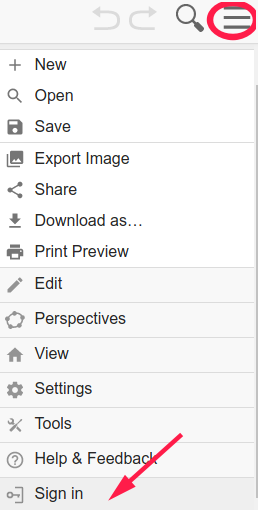• 右上角三字形状的图标--->Share, 弹出一个窗口, 可以选择 Shared(分享) 或者 Private(私密), 这个可以根据需求选择, 然后点 SAVE(保存), 会弹出一个对话框, 里面有一个链接, 可以复制这个链接, 然后分享给他人